# 1750 and Level 1

### Today’s Puzzle:

Place the numbers 1 to 12 in the first column and also in the top row so that those numbers are the factors of the given clues.### Factors of 1750:

• 1750 is a composite number.
• Prime factorization: 1750 = 2 × 5 × 5 × 5 × 7, which can be written 1750 = 2 × 5³ × 7.
• 1750 has at least one exponent greater than 1 in its prime factorization so √1750 can be simplified. Taking the factor pair from the factor pair table below with the largest square number factor, we get √1750 = (√25)(√70) = 5√70.
• The exponents in the prime factorization are 1,3 and 1. Adding one to each exponent and multiplying we get (1 + 1)(3 + 1)(1 + 1) = 2 × 4 × 2 = 16. Therefore 1750 has exactly 16 factors.
• The factors of 1750 are outlined with their factor pair partners in the graphic below.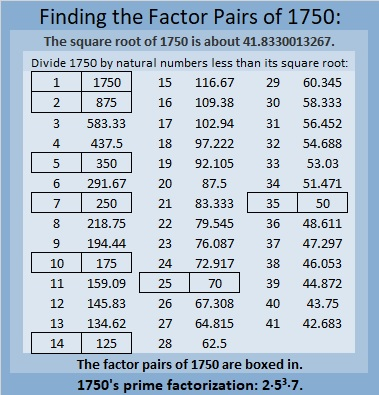### More About the Number 1750:

1750 is the hypotenuse of THREE Pythagorean triples:
490 1680 1750, which is (7-24-25) times 70,
616 1638 1750, which is 14 times (44-117-125), and
1050 1400 1750, which is (3-4-5) times 350.

1750 looks interesting in some other bases:
It’s 5050 in base 7 because 5(7³) + 5(7) = 1750, and it’s
1K1 in base 33 because 1(33²) + 20(33) + 1(1) = 1750.

# 1739 A Gift for a Valentine

### Today’s Puzzle:

Here’s a level 1 Valentine’s gift puzzle to start off the month of February. Place the numbers 1 to 10 in both the first column and the top row so that those numbers are the factors of the given number clues.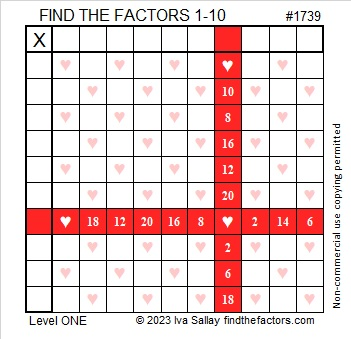And here’s a heartless copy of the same puzzle that may be more printer-friendly.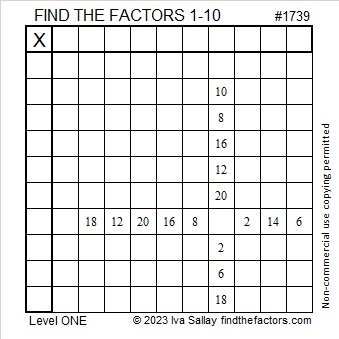### Factors of 1739:

Is 1739 a prime number? It isn’t divisible by 2 or 5.
1 + 7 + 3 + 9 = 20, so it isn’t divisible by 3.
1 – 7 + 3 – 9 = -12, so it isn’t divisible by 11.
√1739 is a little more than 41. Should I try dividing 1739 by every other prime number less than 41? I don’t know divisibility tricks for most of those prime numbers!

Here’s a shortcut I first wrote about in 341 is the smallest composite number that gives a false positive for this Quick Prime Number Test: First I type into my computer’s scientific calculator:
2  xʸ  1739  Mod  1739  =

If the answer on the calculator is anything other than “2”, then it can’t be a prime number. If it is “2”, it very likely is prime. As you can see by the screenshot of my calculator, 1739 is not prime!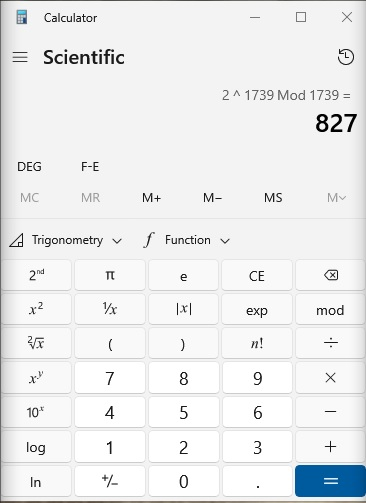• 1739 is a composite number.
• Prime factorization: 1739 = 37 × 47.
• 1739 has no exponents greater than 1 in its prime factorization, so √1739 cannot be simplified.
• The exponents in the prime factorization are 1 and 1. Adding one to each exponent and multiplying we get (1 + 1)(1 + 1) = 2 × 2 = 4. Therefore 1739 has exactly 4 factors.
• The factors of 1739 are outlined with their factor pair partners in the graphic below.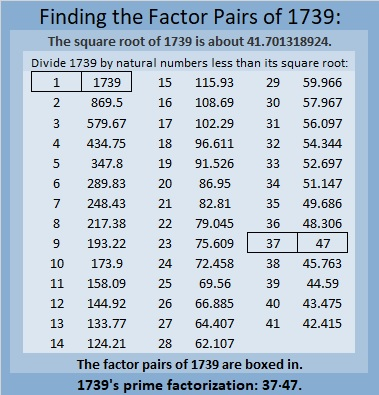### More About the number 1739:

1739 is the difference of two squares in two ways:
870² – 869² = 1739, and
42² – 5² = 1739.

That means 1739 is only 25 numbers away from the next perfect square!

# How Is 1730 the Sum of Consecutive Squares?

### Today’s Puzzle:

Write all the numbers from 1 to 12 in both the first column and the top row so that those numbers are the factors of the given clues.### Factors of 1730:

• 1730 is a composite number.
• Prime factorization: 1730 = 2 × 5 × 173.
• 1730 has no exponents greater than 1 in its prime factorization, so √1730 cannot be simplified.
• The exponents in the prime factorization are 1, 1, and 1. Adding one to each exponent and multiplying we get (1 + 1)(1 + 1)(1 + 1) = 2 × 2 × 2 = 8. Therefore 1730 has exactly 8 factors.
• The factors of 1730 are outlined with their factor pair partners in the graphic below.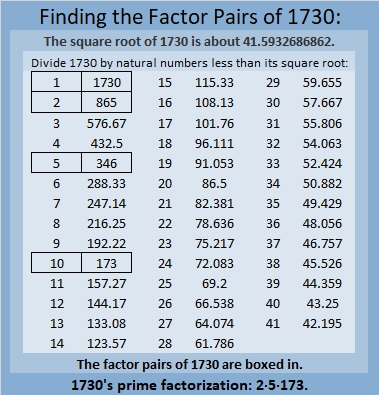### More about the number 1730:

1730 is the sum of two squares in two different ways:
41² + 7² = 1730, and
37² + 19² = 1730.

1730 is the hypotenuse of FOUR Pythagorean triples:
520 1650 1730 which is 10 times (52-165-173)
574 1632 1730 calculated from 2(41)( 7), 41² – 7², 41² + 7²,
1008 1406 1730 calculated from 37² – 19², 2(37)(19), 37² + 19², and
1038 1384 1730 which is 346 times (3-4-5).

Finally, OEIS.org informs us that 1730 is the sum of consecutive squares in two different ways. What are those two ways? I figured it out. Can you?

Here’s a hint: It is the sum of three consecutive squares as well as twelve consecutive squares. That means √(1730/3) rounded is included in one sum and √(1730/12) rounded is included in the other. The solution can be found in the comments. Have fun finding them yourself though!

# 1721 A Gift With Multiple Treasures

### Today’s Puzzle:

‘Tis the season of giving, and here’s a gift with multiple treasures inside. Write the numbers from 1 to 10 in both the first column and the top row so that the given clues are the products of the numbers you write. After you find the factors, you can complete the puzzle by finding all of the products.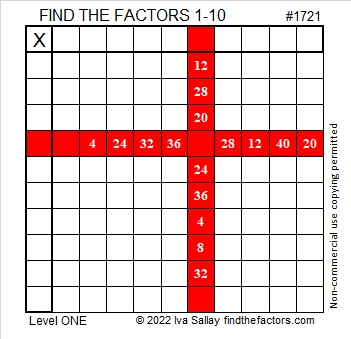### Factors of 1721:

• 1721 is a prime number.
• Prime factorization: 1721 is prime.
• 1721 has no exponents greater than 1 in its prime factorization, so √1721 cannot be simplified.
• The exponent in the prime factorization is 1. Adding one to that exponent we get (1 + 1) = 2. Therefore 1721 has exactly 2 factors.
• The factors of 1721 are outlined with their factor pair partners in the graphic below.How do we know that 1721 is a prime number? If 1721 were not a prime number, then it would be divisible by at least one prime number less than or equal to √1721. Since 1721 cannot be divided evenly by 2, 3, 5, 7, 11, 13, 17, 19, 23, 29, 31, 37, or 41, we know that 1721 is a prime number.

### More About the Number 1721:

1721 is the sum of two squares:
40² + 11² = 1721.

Here’s another way we know that 1721 is a prime number: Since its last two digits divided by 4 leave a remainder of 1, and 40² + 11² = 1721 with 40 and 11 having no common prime factors, 1721 will be prime unless it is divisible by a prime number Pythagorean triple hypotenuse less than or equal to √1721. Since 1721 is not divisible by 5, 13, 17, 29, 37, or 41, we know that 1721 is a prime number.

1721 and 1723 are twin primes.

# 1696 Inverses

### Today’s Puzzle:

Joseph Nebus of Nebusresearch recently wrote an extensive post about inverses at my request. Although inverses are often fascinating, advanced topics in mathematics, they can also be quite simple. For example, solving this puzzle will involve using the inverse of multiplication, division, AND it is the simplest division possible this time. Since it is December, I made this puzzle look like a gift for you. Just write the numbers 1 to 12 in both the first column and the top row so that those numbers and the given clues could become a multiplication table.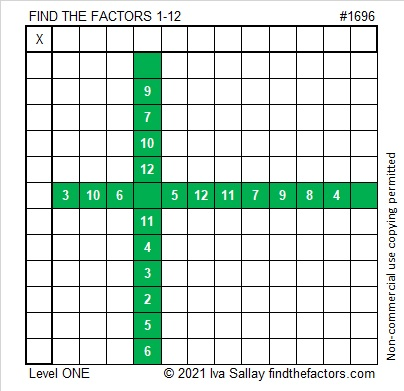If you want to print the puzzle without color, here it is:### Factors of 1696:

• 1696 is a composite number.
• Prime factorization: 1696 = 2 × 2 × 2 × 2 × 2 × 53, which can be written 1696 = 2⁵ × 53.
• 1696 has at least one exponent greater than 1 in its prime factorization so √1696 can be simplified. Taking the factor pair from the factor pair table below with the largest square number factor, we get √1696 = (√16)(√106) = 4√106.
• The exponents in the prime factorization are 5 and 1. Adding one to each exponent and multiplying we get (5 + 1)(1 + 1) = 6 × 2 = 12. Therefore 1696 has exactly 12 factors.
• The factors of 1696 are outlined with their factor pair partners in the graphic below.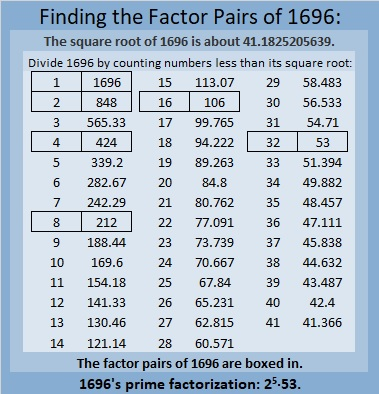### More About the Number 1696:

1696 is the sum of two squares:
36² + 20² = 1696.

1696 is also the hypotenuse of a Pythagorean triple:
896-1440 -1696, which is 32 times (28-45-52).
It can also be calculated from 36² – 20², 2(36)(20), 36² + 20².

1696 is also the difference of two squares in FOUR different ways:
425² – 423² = 1696,
214² – 210² = 1696,
110² – 102² = 1696, and
61² – 45² = 1696.
I found those equations by using the even factor pairs of 1696 and taking the inverse of the fact that a² – b² = (a + b)(a – b).

# 1683 Grave Marker

### Today’s Puzzle:

It’s almost Halloween. I hope you enjoy this grave-marker puzzle. Write the numbers from 1 to 12 in both the first column and the top row so that those numbers and the given clues make a multiplication table.Here’s the same puzzle, but it won’t use up all your printer ink.### Factors of 1683:

• 1683 is a composite number.
• Prime factorization: 1683 = 3 × 3 × 11 × 17, which can be written 1683 = 3² × 11 × 17.
• 1683 has at least one exponent greater than 1 in its prime factorization so √1683 can be simplified. Taking the factor pair from the factor pair table below with the largest square number factor, we get √1683 = (√9)(√187) = 3√187.
• The exponents in the prime factorization are 2, 1, and 1. Adding one to each exponent and multiplying we get (2 + 1)(1 + 1)(1 + 1) = 3 × 2 × 2 = 12. Therefore 1683 has exactly 12 factors.
• The factors of 1683 are outlined with their factor pair partners in the graphic below.### More About the Number 1683:

1683 is the hypotenuse of a Pythagorean triple:
792-1485-1683, which is (8-15-17) times 99.

1683 is the difference of two squares in SIX different ways:
842² – 841² = 1683,
282² – 279² = 1683,
98² – 89² = 1683,
82² – 71² = 1683,
58² – 41² = 1683, and
42² – 9² = 1683.
That last one means we are 81 numbers away from the next perfect square. I also highlighted a cool-looking difference.

1680, 1681, 1682, 1683, and 1684 are the second smallest set of FIVE consecutive numbers whose square roots can be simplified.# 1673 and Level 1

### Today’s Puzzle:

Write the numbers from 1 to 10 in both the first column and the top row so that those numbers and the given clues become a not-in-the-usual-order multiplication table.### Factors of 1673:

• 1673 is a composite number.
• Prime factorization: 1673 = 7 × 239.
• 1673 has no exponents greater than 1 in its prime factorization, so √1673 cannot be simplified.
• The exponents in the prime factorization are 1 and 1. Adding one to each exponent and multiplying we get (1 + 1)(1 + 1) = 2 × 2 = 4. Therefore 1673 has exactly 4 factors.
• The factors of 1673 are outlined with their factor pair partners in the graphic below.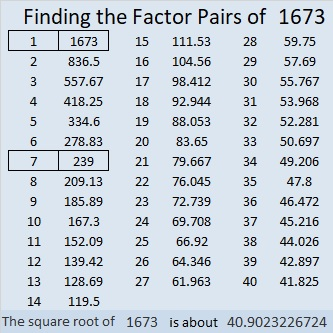### More About the Number 1673:

The factors of 1673 are 1, 7, 239, and 1673. OEIS.org tells us something cool about the sum of the squares of those factors:
1² + 7² + 239² + 1673² = 1690².

# 1663 and Level 1

### Today’s Puzzle:

Write the numbers 1 to 12 in both the first column and the top row so that those numbers and the given clues function like a multiplication table.### Factors of 1663:

• 1663 is a prime number.
• Prime factorization: 1663 is prime.
• 1663 has no exponents greater than 1 in its prime factorization, so √1663 cannot be simplified.
• The exponent in the prime factorization is 1. Adding one to that exponent we get (1 + 1) = 2. Therefore 1663 has exactly 2 factors.
• The factors of 1663 are outlined with their factor pair partners in the graphic below.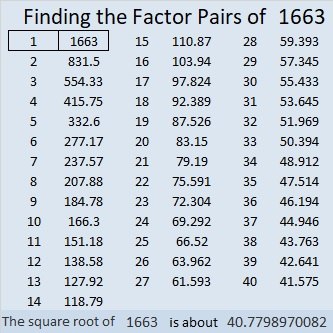How do we know that 1663 is a prime number? If 1663 were not a prime number, then it would be divisible by at least one prime number less than or equal to √1663. Since 1663 cannot be divided evenly by 2, 3, 5, 7, 11, 13, 17, 19, 23, 29, 31, or 37, we know that 1663 is a prime number.

### More About the Number 1663:

1663 is the sum of consecutive numbers in only one way:
831 + 832 = 1663.

1663 is the difference of two squares in only one way:
832² – 831² = 1663.

What do you notice about those two number facts?

# 1650 Wrinkles in the Multiplication Table

### Today’s Puzzle:

Are you familiar with the book A Wrinkle in Time? Kat of The Lily Cafe’s blog loves books and recently compared Meg in that book to her six-year-old son. She wrote a post titled Am I Raising a Meg? Her six-year-old LOVES math and is very much interested in multiplication and division. When Mom thought he was playing a game on her phone, he was actually playing with the calculator app! I felt so happy inside as I read that!

I wonder if they have discovered the storybooks in the Math Book Magic blog. Such books could combine Mom’s love for reading with her son’s love of math.

Someday her son might like to solve a “wrinkled” multiplication table puzzle like this one that has only nine clues.

Write all the numbers 1 to 10 in both the first column and the top row so that those numbers and the given clues become a multiplication table.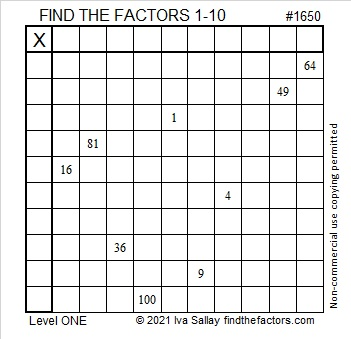### Factor Cake for 1650:

This is my 1650th post.
1650 is divisible by 2 and by 5 because it ends with a 0.
1650 is divisible by 3 because 1 + 6 + 5 + 0 = 12, a number divisible by 3.
1650 is divisible by 11 because 1 – 6 + 5 – 0 = 0, a number divisible by 11.

I think we can make a lovely factor cake for 1650: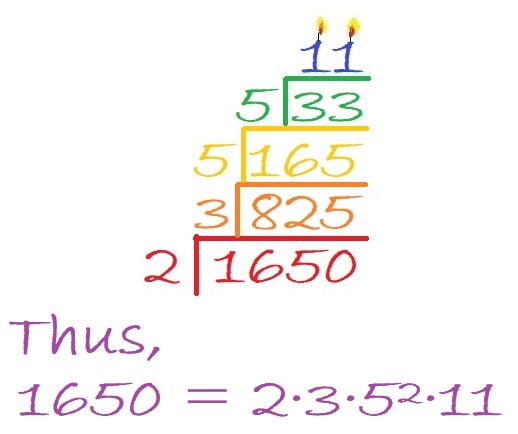### Factors of 1650:

• 1650 is a composite number.
• Prime factorization: 1650 = 2 × 3 × 5 × 5 × 11, which can be written 1650 = 2 × 3 × 5² × 11.
• 1650 has at least one exponent greater than 1 in its prime factorization so √1650 can be simplified. Taking the factor pair from the factor pair table below with the largest square number factor, we get √1650 = (√25)(√66) = 5√66.
• The exponents in the prime factorization are 1, 1, 2, and 1. Adding one to each exponent and multiplying we get (1 + 1)(1 + 1)(2 + 1)(1 + 1) = 2 × 2 × 3 × 2 = 24. Therefore 1650 has exactly 24 factors.
• The factors of 1650 are outlined with their factor pair partners in the graphic below.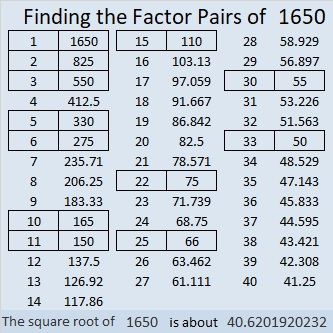### More About the Number 1650:

1650 is the hypotenuse of TWO Pythagorean triples:
462-1584-1650, which is (7-24-25) times 66, and
990-1320-1650, which is (3-4-5) times 330.

# 1639 and Level 1

### Today’s Puzzle:

Write the numbers from 1 to 12 in both the first column and the top row so that those numbers and the given clues will make this puzzle function like a multiplication table.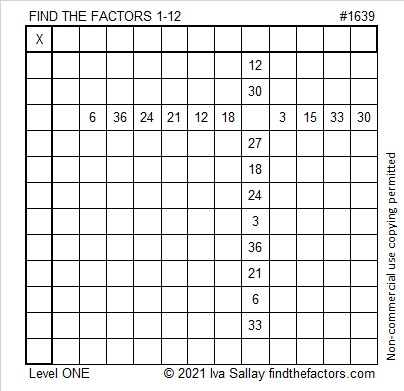### Factors of 1639:

1 – 6 + 3 – 9 = -11 so 1639 is divisible by 11.

• 1639 is a composite number.
• Prime factorization: 1639 = 11 × 149.
• 1639 has no exponents greater than 1 in its prime factorization, so √1639 cannot be simplified.
• The exponents in the prime factorization are 1 and 1. Adding one to each exponent and multiplying we get (1 + 1)(1 + 1) = 2 × 2 = 4. Therefore 1639 has exactly 4 factors.
• The factors of 1639 are outlined with their factor pair partners in the graphic below.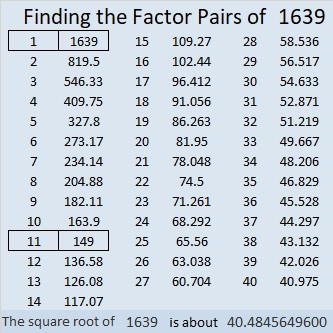### More about the Number 1639:

1639 is the hypotenuse of a Pythagorean triple:
561 1540 1639, which is 11 times (51-140-149).

1639 is the 22nd nonagonal number because
22(7·22 – 5)/2 =
22(154 – 5)/2=
22(149)/2 =
11(149) = 1639.
Mathworld.Wolfram has illustrations of the first 5 nonagonal numbers.